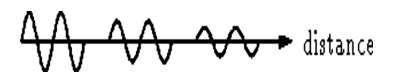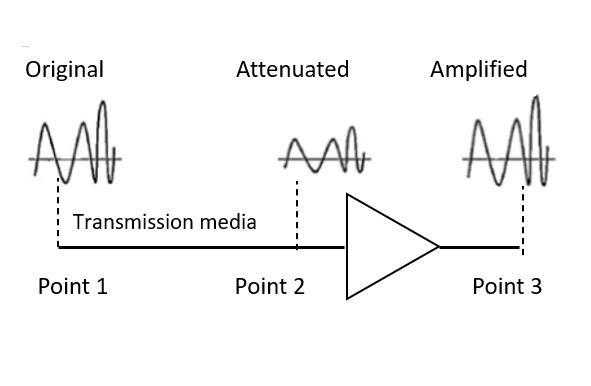# What is Attenuation Distortion in computer networks?

Computer NetworkInternetMCA

Transmission impairment occurs when the received signal is different from the transmitted signal. As we know, a signal can be transmitted as Analog signal or it can be transmitted as a digital signal.

In Analog signals due to transmission impairment the resulting received signal gets different amplitude or the shape. In the case of digitally transmitted signals at the receiver side we get changes in bits (0's or 1's).

## Causes

There are various causes of transmission impairments, which are as follows −

• Noise
• Delay Distortion
• Attenuation Distortion

Let us discuss Attenuation Distortion.

## Attenuation Distortion

Attenuation is generally decreased in signal strength, by which the received signal will be difficult to receive at the receiver end. This attenuation happens due to the majority factor by environment as the environment imposes a lot of resistance and the signal strength decreases as it tries to overcome the resistance imposed.The above picture shows that the signal loses power at its travel time.In the above diagram it is clear when a signal is transmitting from point 1 to point 2, due to Attenuation distortion the signal loses its power at its travel time before it reaches the destination.

So to overcome this distortion we can use an amplifier, to get the original signal at the receiver side also.

## Issues

The issues in Attenuation Distortion are as follows −

• Signals have to be strong so that the receiver can detect and interpret them.

• They should maintain a sufficient high level to make them differentiate from noise

• Even the too strong signals can also overload the circuitry of the transmitter and result in distortion.

• They should consider that attenuation increases with frequency.

## Measuring Attenuation

Attenuation is measured in Bel as follows −

$$\mathrm{log_{10}\frac{Power_{in}}{Power_{out}}\:Bel}$$

The above formula can also be represented in decibel (dB) as follows −

$$\mathrm{20^*log_{10}\frac{Power_{in}}{Power_{out}}\:Decibel}$$

Decibels are used because of the following −

• Logarithmically Signal strengths fall off.

• Cascade gains and losses are calculated with simple additions and subtractions.